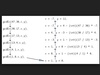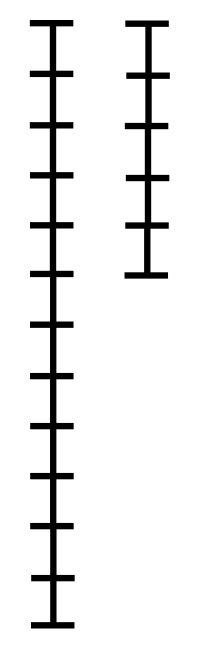gcd(a,b) = gcd(b,a mod b)

Euclidean Algorithm 或者 Euclid's algorithm

• 辗转相除法 辗转相除法相信大家大学经常接触，但初次接触到可能不是那么容易理解，那么话不多说，我跟大家分享一下我的理解。 关于辗转相除法的专业解释有很多，我在这就不再赘述，大家可以自己搜搜看，我这直接上...
辗转相除法
关于辗转相除法的专业解释有很多，我在这就不再赘述，大家可以自己搜搜看，我这直接上图。辗转相除法计算的是	a 和 b 的 最大公约数 g
这里先将 a 分成两份 分别由 b 和 c 组成
a % b = c

再求 b 和 c 的最大公约数 g
 g = gcd(b,c)

这时b / g = 0  , c / g = 0
由于a可以由 b 和 c 组成，
所以  a / g = 0;
可得 a  和 b 的最大公约数为 g
这里举两个简单的例子：
a = 10, b = 8;
a % b = 2;
c = 2;
a 被分为了 8 和 2 两部分；
因为 8 和 2 的最大公约数为 2
而 a 由 b 和 c 组成
10 = 8 + 2 = 4 * 2 + 2 = 5 * 2
所以10和8的最大公约数为2；
再例如：
a = 18, b = 8
c = a % b = 2
8 % 2 = 0
18 = 8 * 2 + 2 = 9 * 2
所以 18 和 8 最大公约数为2
具体实现是一个简单的递归调用：
int gcd(int a, int b)
{
if(b == 0) return a;
return gcd(b, a%b);
}

还有一点值得说的是， 一般情况下调用 gcd(a,b) 都是默认 a > b;
不过如果a < b也不用但心，因为当 a < b 时，gcd(a,b) = gcd(b,a%b) = gcd(b,a)


展开全文c++ c语言 后端
• 辗转相除法证明辗转相除法证明辗转相除法证明辗转相除法证明...